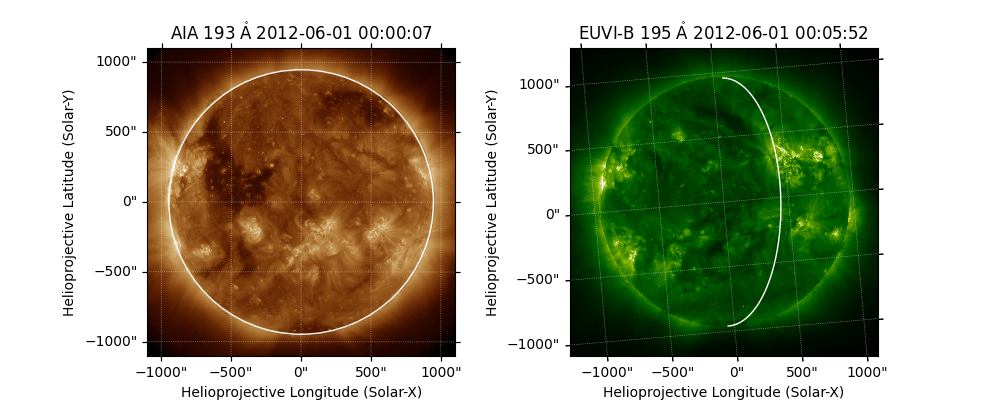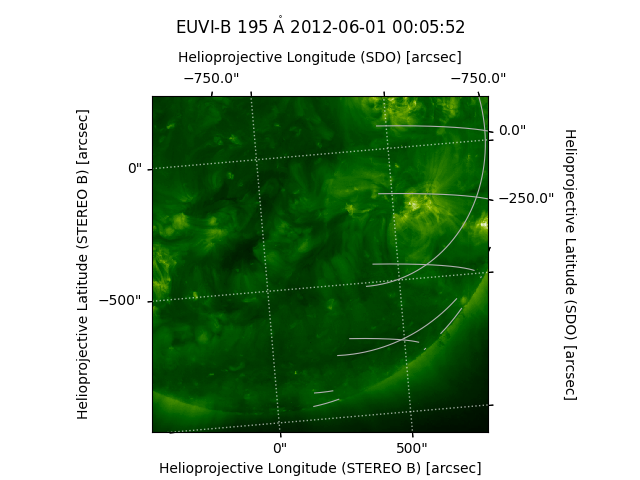# Drawing the AIA limb on a STEREO EUVI image¶

In this example we use a STEREO-B and an SDO image to demonstrate how to overplot the limb as seen by AIA on an EUVI-B image. Then we overplot the AIA coordinate grid on the STEREO image.

import matplotlib.pyplot as plt

import astropy.units as u
from astropy.coordinates import SkyCoord

import sunpy.coordinates.wcs_utils
import sunpy.map
from sunpy.net import Fido
from sunpy.net import attrs as a


The first step is to download some data. We download an image from STEREO-A and an image from SDO, which are separated in longitude.

stereo = (a.Source('STEREO_A') &
a.Instrument("EUVI") &
a.Time('2021-01-01 00:06', '2021-01-01 00:07'))

aia = (a.Instrument.aia &
a.Sample(24 * u.hour) &
a.Time('2021-01-01 00:06', '2021-01-02 00:06'))

wave = a.Wavelength(30 * u.nm, 31 * u.nm)
result = Fido.search(wave, aia | stereo)


print(result)


Out:

Results from 2 Providers:

1 Results from the VSOClient:
Source: http://vso.stanford.edu/cgi-bin/search
Total estimated size: 67.789 Mbyte

Start Time       ...
...
----------------------- ...
2021-01-01 00:06:05.000 ...

1 Results from the VSOClient:
Source: http://vso.stanford.edu/cgi-bin/search
Total estimated size: 8.41 Mbyte

Start Time               End Time        ...             Info
...
----------------------- ----------------------- ... ----------------------------
2021-01-01 00:06:15.000 2021-01-01 00:06:19.000 ... EUVI ;  ; NORMAL ; 2048x2048

['/home/docs/sunpy/data/aia_lev1_304a_2021_01_01t00_06_05_13z_image_lev1.fits', '/home/docs/sunpy/data/20210101_000615_n4eua.fts']


Let’s create a dictionary with the two maps, which we crop to full disk.

maps = {m.detector: m.submap(SkyCoord([-1100, 1100], [-1100, 1100],
unit=u.arcsec, frame=m.coordinate_frame))
maps['AIA'].plot_settings['vmin'] = 0  # set the minimum plotted pixel value


Out:

INFO: Missing metadata for solar radius: assuming the standard radius of the photosphere. [sunpy.map.mapbase]


Now, let’s plot both maps, and we draw the limb as seen by AIA onto the EUVI image. We remove the part of the limb that is hidden because it is on the far side of the Sun from STEREO’s point of view.

fig = plt.figure(figsize=(10, 4))
maps['AIA'].plot(axes=ax1)
maps['AIA'].draw_limb(axes=ax1)

maps['EUVI'].plot(axes=ax2)
visible, hidden = maps['AIA'].draw_limb(axes=ax2)
hidden.remove()Out:

INFO: Missing metadata for solar radius: assuming the standard radius of the photosphere. [sunpy.map.mapbase]


Let’s plot the helioprojective coordinate grid as seen by SDO on the STEREO image in a cropped view. Note that only those grid lines that intersect the edge of the plot will have corresponding ticks and tick labels.

fig = plt.figure()

maps['EUVI'].plot(axes=ax)

# Crop the view using pixel coordinates
ax.set_xlim(500, 1300)
ax.set_ylim(100, 900)

# Shrink the plot slightly and move the title up to make room for new labels.
ax.set_position([0.1, 0.1, 0.8, 0.7])

# Change the default grid labels and line properties.
stereo_x, stereo_y = ax.coords
stereo_x.set_axislabel("Helioprojective Longitude (STEREO B) [arcsec]")
stereo_y.set_axislabel("Helioprojective Latitude (STEREO B) [arcsec]")
ax.coords.grid(color='white', linewidth=1)

# Add a new coordinate overlay in the SDO frame.
overlay = ax.get_coords_overlay(maps['AIA'].coordinate_frame)
overlay.grid()

# Configure the grid:
x, y = overlay

# Wrap the longitude at 180 deg rather than the default 360.
x.set_coord_type('longitude', 180.)

# Set the tick spacing
x.set_ticks(spacing=250*u.arcsec)
y.set_ticks(spacing=250*u.arcsec)

# Set the ticks to be on the top and left axes.
x.set_ticks_position('tr')
y.set_ticks_position('tr')

# Change the defaults to arcseconds
x.set_major_formatter('s.s')
y.set_major_formatter('s.s')